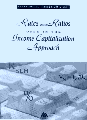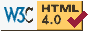## Rates and Ratios Used in the Income Capitalization Approach

by Clifford Fisher Jr., MAI

#### Review by Stephen Traub, ASABEGINNING WHERE ITS predecessor, Mathematics for Real Estate Appraisers, leaves off, this booklet focuses exclusively on the math used in the income capitalization approach. It is a handy reference that can be used similarly to the way in which one would look up a word in a dictionary. Not only will one get a description of the many rates, ratios, and techniques, but also one will get examples and their accompanying caveats.

"Too often, a method or technique is misused, or even worse, an appropriate method ... is deemed invalid. This handbook was written to prevent these types of scenario from occurring," states the author.

One check for "reasonableness" is to compare the overall yield rate, `Y(O)`, to the mortgage interest rate. If the interest rate is higher, the appraiser should check his/her calculations, rethink his/her reasoning, or if left as is, should provide a convincing explanation for this unusual situation.

The booklet contains four parts: 1) background; 2) frequently used rates and ratios, common names, symbols, and their relationship to other rates; 3) traditional extraction techniques and frequently misunderstood aspects; and 4) non-traditional, but legitimate techniques.

### Rates

Common rates on the whole property include `R(O)` also depicted as `OAR` or `Y(O)`. Rates, however, may represent just a component of the whole: financial components include those on the mortgage component, `R(M)`; the equity component, `R(E)`; and yields, `Y(M)` and `Y(E)`. Legal components include: the yield of the leased fee component, `Y(LF)`; the leasehold component, `Y(LH)`; and the sub-leasehold (sandwich), `R(SAND1)`.

The `OAR` or `R(O)` is derived with the formula `I(O)/V(O) = R(O)`. This is different from the equity cap rate component also known as the equity dividend rate, or cash-on-cash rate, or cash-flow rate, `R(E)` (though without financing, `R(E)` will equal `R(O)`). `R(E)` can be extracted from a sale using the formula, `I(E)/V(E) = R(E)`. `R(E)`, however, should be extracted before a cash equivalency adjustment.

The yield rate, `Y(E)`, on the other hand (also known as the risk rate, "return on" rate, `IRR`, or discount rate), considers more than just the first year cash return. It considers all cash attributable to the equity investment over a holding period including proceeds from sale at termination

In a case in which discounted cash flow analysis is applied, resale value at the end of the holding period is calculated using a "going out" cap rate, `R(N)`. `R(N)` rarely will be less than the "going in" rate, `R(O)`, because investors typically factor in the uncertainty for time and improvements will be closer to the end of their economic life.### Ratios

Common ratios include loan-to-value (`M`), debt coverage ratios (`DCR`), and operating expense ratios (`OER`). `DCR` is calculated by comparing a property's annual net operating income (`NOI`) to its debt service costs. It measures the ability to meet the mortgage payments from the `NOI`, so the `DCR` is usually required to be greater than 1.0. `DCR` can be extracted by dividing the `NOI` by the annual debt service costs. It is commonly seen in the following formula: `R(O) = M * R(M) * DCR`.

The expense ratio or `OER` is the total operating expenses divided by the effective gross income or `TOE/EGI`. The `OER` is useful in testing the "reasonableness" of the operating expenses estimated in a reconstructed operating statement. Benchmark `OER`s can be derived from an analysis of similar properties.

### Techniques

In Part 3, extraction techniques include a simple extraction of an `R(O)` from a sale. The author also illustrates the extraction of an equity dividend rate, `R(E)`.

To extract the `R(E)`, debt service costs are subtracted from the `NOI`. The difference is divided by the equity amount, resulting in the equity dividend rate, or cash-on-cash rate. In formulaic terms this is written as:

`NOI - Debt Service Costs = I(E)`

`I(E)/V(E) = R(E)`

In the final chapter, Chapter 4, the booklet closes with examples of more complex, two variable extractions (similar to sensitivity analysis) with an example of the results of a two variable extraction illustrated below:

Change
Rate
-- Y(O)
-2% -- 10%
0% -- 12%
+2% -- 14%

If you like brief, simple, and handy reference booklets, Rates and Ratios Used in the Income Capitalization Approach will rate highly with you.

The book above is available on-line at Amazon Books.

Stephen Traub, ASA, the reviewer, is chief commercial appraiser for Property Valuation Advisors, 63 Hill St., Newburyport, MA 01950. He is a certified general appraiser in NH, ME and MA.

To contact the author of this review, e-mail to:straub@shore.net or contact him at the address above, or call 978-462-4347.Copyright © 1996-97 Property Valuation Advisors, Newburyport, MA. All rights reserved. Disclaimer